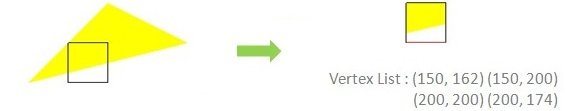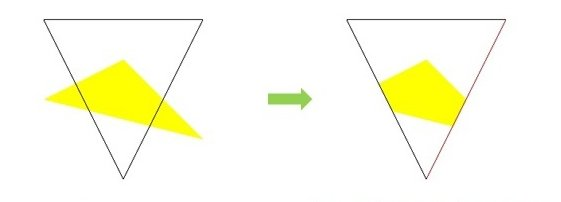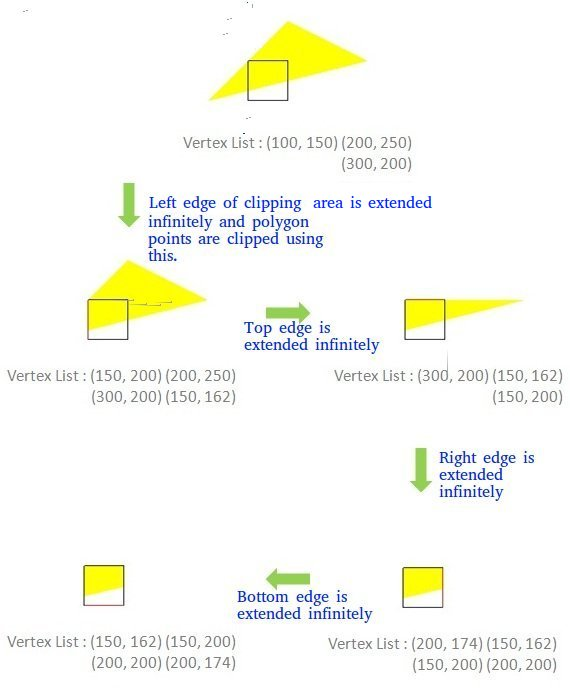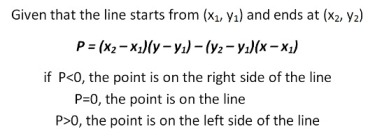# Polygon Clipping | Sutherland–Hodgman Algorithm

• Difficulty Level : Hard
• Last Updated : 15 May, 2017

A convex polygon and a convex clipping area are given. The task is to clip polygon edges using the Sutherland–Hodgman Algorithm. Input is in the form of vertices of the polygon in clockwise order.

Examples:

Attention reader! Don’t stop learning now. Get hold of all the important DSA concepts with the DSA Self Paced Course at a student-friendly price and become industry ready.  To complete your preparation from learning a language to DS Algo and many more,  please refer Complete Interview Preparation Course.

In case you wish to attend live classes with experts, please refer DSA Live Classes for Working Professionals and Competitive Programming Live for Students.

```Input : Polygon : (100,150), (200,250), (300,200)
Clipping Area : (150,150), (150,200), (200,200),
(200,150) i.e. a Square
Output : (150, 162) (150, 200) (200, 200) (200, 174)Example 2
Input : Polygon : (100,150), (200,250), (300,200)
Clipping Area : (100,300), (300,300), (200,100)
Output : (242, 185) (166, 166) (150, 200) (200, 250) (260, 220)```

## Recommended: Please try your approach on {IDE} first, before moving on to the solution.

Overview of the algorithm:

```Consider each edge e of clipping Area  and do following:
a) Clip given polygon against e.
```

How to clip against an edge of clipping area?
The edge (of clipping area) is extended infinitely to create a boundary and all the vertices are clipped using this boundary. The new list of vertices generated is passed to the next edge of the clip polygon in clockwise fashion until all the edges have been used.

There are four possible cases for any given edge of given polygon against current clipping edge e.

1. Both vertices are inside : Only the second vertex is added to the output list
2. First vertex is outside while second one is inside : Both the point of intersection of the edge with the clip boundary and the second vertex are added to the output list
3. First vertex is inside while second one is outside : Only the point of intersection of the edge with the clip boundary is added to the output list
4. Both vertices are outside : No vertices are added to the output listThere are two sub-problems that need to be discussed before implementing the algorithm:-

To decide if a point is inside or outside the clipper polygon
If the vertices of the clipper polygon are given in clockwise order then all the points lying on the right side of the clipper edges are inside that polygon. This can be calculated using :To find the point of intersection of an edge with the clip boundary
If two points of each line(1,2 & 3,4) are known, then their point of intersection can be calculated using the formula :-`// C++ program for implementing Sutherland–Hodgman``// algorithm for polygon clipping``#include``using` `namespace` `std;`` ` `const` `int` `MAX_POINTS = 20;`` ` `// Returns x-value of point of intersectipn of two``// lines``int` `x_intersect(``int` `x1, ``int` `y1, ``int` `x2, ``int` `y2,``                ``int` `x3, ``int` `y3, ``int` `x4, ``int` `y4)``{``    ``int` `num = (x1*y2 - y1*x2) * (x3-x4) -``              ``(x1-x2) * (x3*y4 - y3*x4);``    ``int` `den = (x1-x2) * (y3-y4) - (y1-y2) * (x3-x4);``    ``return` `num/den;``}`` ` `// Returns y-value of point of intersectipn of``// two lines``int` `y_intersect(``int` `x1, ``int` `y1, ``int` `x2, ``int` `y2,``                ``int` `x3, ``int` `y3, ``int` `x4, ``int` `y4)``{``    ``int` `num = (x1*y2 - y1*x2) * (y3-y4) -``              ``(y1-y2) * (x3*y4 - y3*x4);``    ``int` `den = (x1-x2) * (y3-y4) - (y1-y2) * (x3-x4);``    ``return` `num/den;``}`` ` `// This functions clips all the edges w.r.t one clip``// edge of clipping area``void` `clip(``int` `poly_points[], ``int` `&poly_size,``          ``int` `x1, ``int` `y1, ``int` `x2, ``int` `y2)``{``    ``int` `new_points[MAX_POINTS], new_poly_size = 0;`` ` `    ``// (ix,iy),(kx,ky) are the co-ordinate values of``    ``// the points``    ``for` `(``int` `i = 0; i < poly_size; i++)``    ``{``        ``// i and k form a line in polygon``        ``int` `k = (i+1) % poly_size;``        ``int` `ix = poly_points[i], iy = poly_points[i];``        ``int` `kx = poly_points[k], ky = poly_points[k];`` ` `        ``// Calculating position of first point``        ``// w.r.t. clipper line``        ``int` `i_pos = (x2-x1) * (iy-y1) - (y2-y1) * (ix-x1);`` ` `        ``// Calculating position of second point``        ``// w.r.t. clipper line``        ``int` `k_pos = (x2-x1) * (ky-y1) - (y2-y1) * (kx-x1);`` ` `        ``// Case 1 : When both points are inside``        ``if` `(i_pos < 0  && k_pos < 0)``        ``{``            ``//Only second point is added``            ``new_points[new_poly_size] = kx;``            ``new_points[new_poly_size] = ky;``            ``new_poly_size++;``        ``}`` ` `        ``// Case 2: When only first point is outside``        ``else` `if` `(i_pos >= 0  && k_pos < 0)``        ``{``            ``// Point of intersection with edge``            ``// and the second point is added``            ``new_points[new_poly_size] = x_intersect(x1,``                              ``y1, x2, y2, ix, iy, kx, ky);``            ``new_points[new_poly_size] = y_intersect(x1,``                              ``y1, x2, y2, ix, iy, kx, ky);``            ``new_poly_size++;`` ` `            ``new_points[new_poly_size] = kx;``            ``new_points[new_poly_size] = ky;``            ``new_poly_size++;``        ``}`` ` `        ``// Case 3: When only second point is outside``        ``else` `if` `(i_pos < 0  && k_pos >= 0)``        ``{``            ``//Only point of intersection with edge is added``            ``new_points[new_poly_size] = x_intersect(x1,``                              ``y1, x2, y2, ix, iy, kx, ky);``            ``new_points[new_poly_size] = y_intersect(x1,``                              ``y1, x2, y2, ix, iy, kx, ky);``            ``new_poly_size++;``        ``}`` ` `        ``// Case 4: When both points are outside``        ``else``        ``{``            ``//No points are added``        ``}``    ``}`` ` `    ``// Copying new points into original array``    ``// and changing the no. of vertices``    ``poly_size = new_poly_size;``    ``for` `(``int` `i = 0; i < poly_size; i++)``    ``{``        ``poly_points[i] = new_points[i];``        ``poly_points[i] = new_points[i];``    ``}``}`` ` `// Implements Sutherland–Hodgman algorithm``void` `suthHodgClip(``int` `poly_points[], ``int` `poly_size,``                  ``int` `clipper_points[], ``int` `clipper_size)``{``    ``//i and k are two consecutive indexes``    ``for` `(``int` `i=0; i

Output:

```(150, 162) (150, 200) (200, 200) (200, 174)
```

This article is contributed by Nabaneet Roy. If you like GeeksforGeeks and would like to contribute, you can also write an article using contribute.geeksforgeeks.org or mail your article to contribute@geeksforgeeks.org. See your article appearing on the GeeksforGeeks main page and help other Geeks.